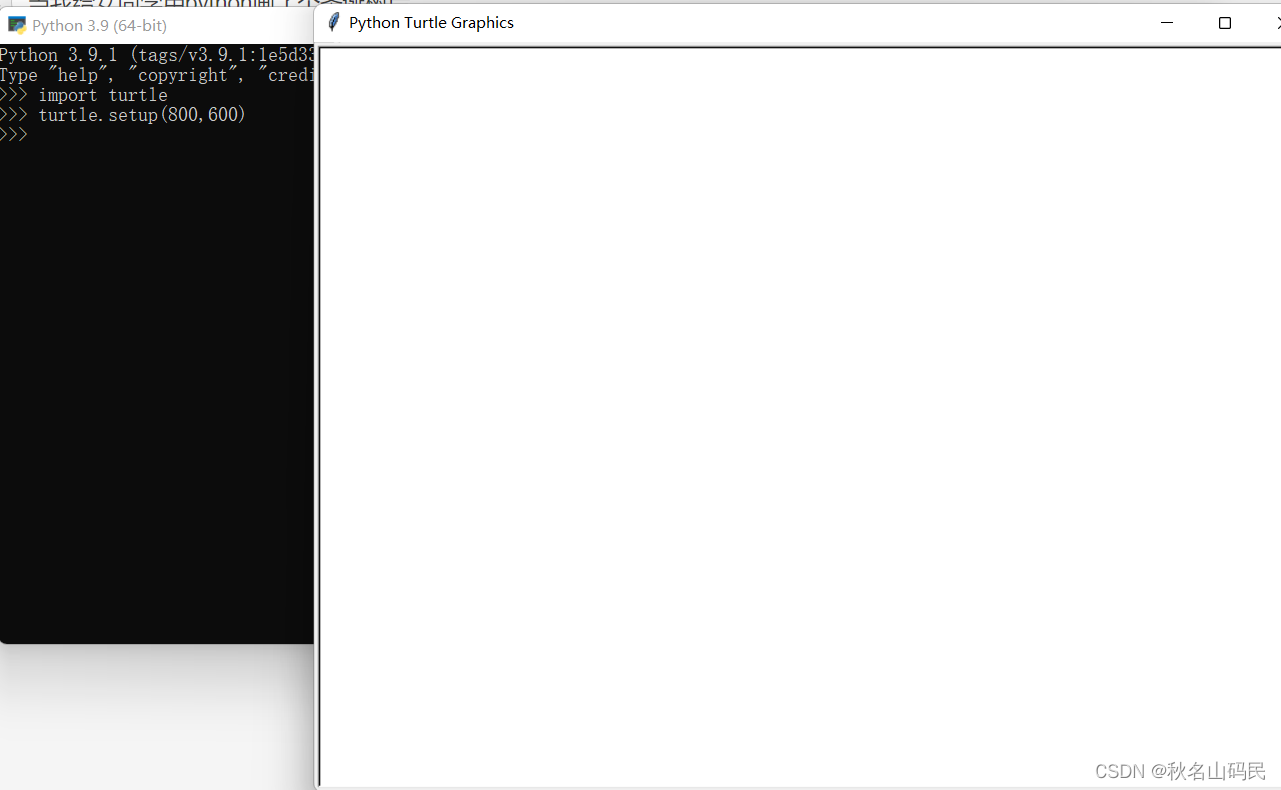﻿ 用python画了个圣诞树给女朋友_python_脚本之家
python# 用python画了个圣诞树给女朋友

## 前言## 开始教学

### 创建

setup（）函数，其中的参数为width，height，startx=None，starty=None，分别表示窗口宽，高，及，窗口在电脑屏幕上的横坐标和纵坐标。

```turtle.setup(800,600)
```### 设置画笔

```pensize(<width>) #粗细
speed(speed) #运动速度
color(color) #设置画笔颜色
```

### 绘制图像

backward(distance) #向后移动

goto(x,y=None)# 移动到相应的位置

4. right(degree)#向右转动
5. left(degree)#向左转动
6. seth(degree)#转动到某个方向

## 正片开始

```from turtle import *
import random #随机，用来搞小圆点
import time

n = 100.0

speed("fastest") #速度快
screensize(bg='seashell')
left(90)
forward(3 * n)
color("orange", "yellow")
begin_fill()
left(126)

for i in range(5):
forward(n / 5)
right(144)
forward(n / 5)
left(72)
end_fill()
right(126)

color("dark green")
backward(n * 4.8)

def tree(d, s):
if d <= 0: return
forward(s)
tree(d - 1, s * .8)
right(120)
tree(d - 3, s * .5)
right(120)
tree(d - 3, s * .5)
right(120)
backward(s)

tree(15, n)
backward(n / 2)

for i in range(200):
a = 200 - 400 * random.random()
b = 10 - 20 * random.random()
up()
forward(b)
left(90)
forward(a)
down()
if random.randint(0, 1) == 0:
color('tomato')
else:
color('wheat')
circle(2)
up()
backward(a)
right(90)
backward(b)

time.sleep(60) #进程推迟时间

```